Examples

Chapter 12 Class 10 Surface Areas and Volumes
Serial order wise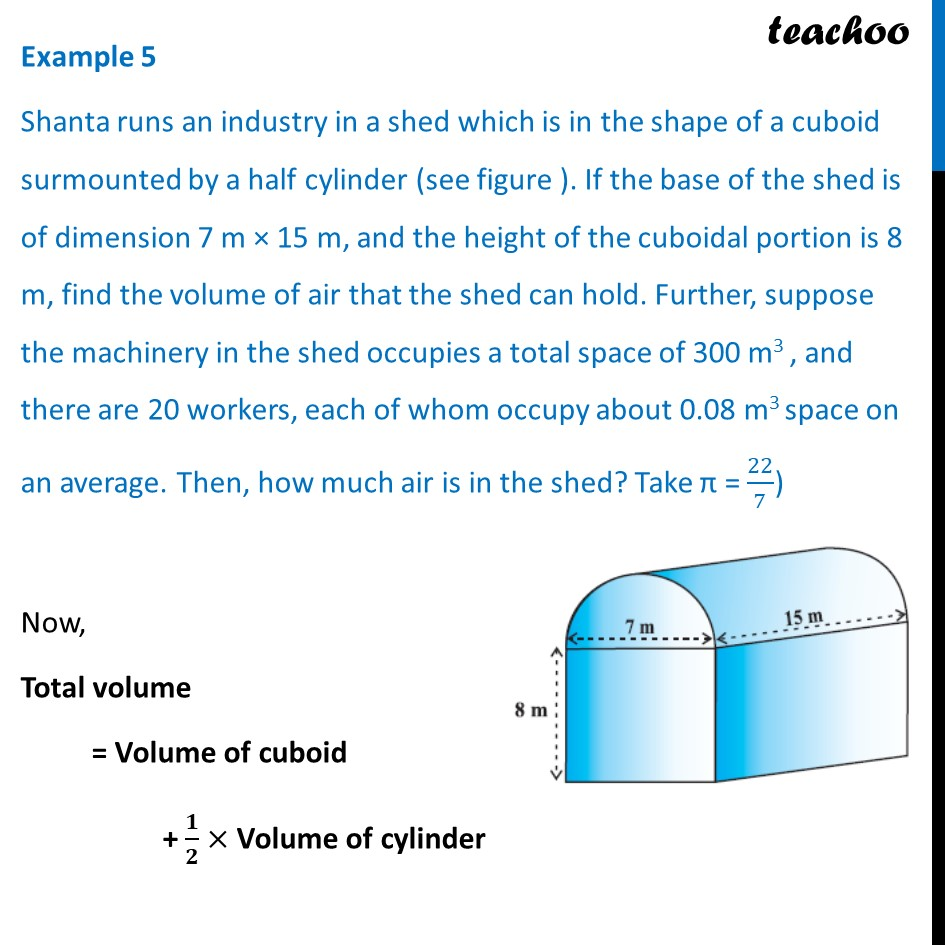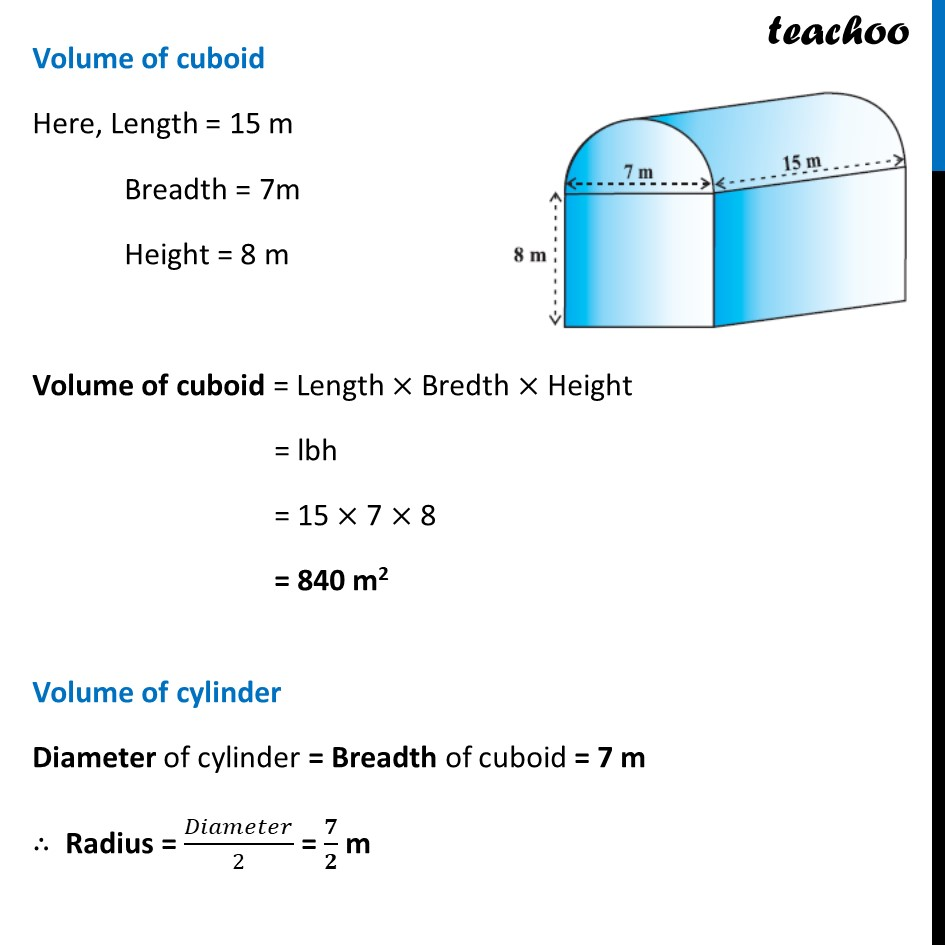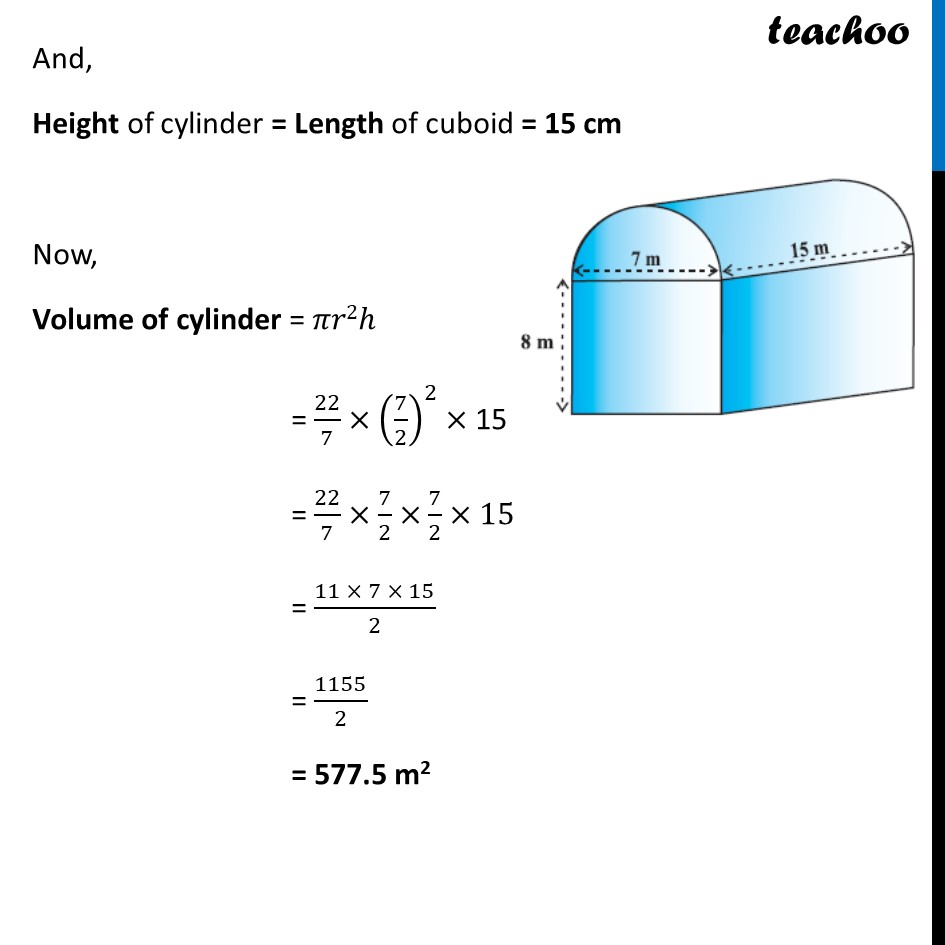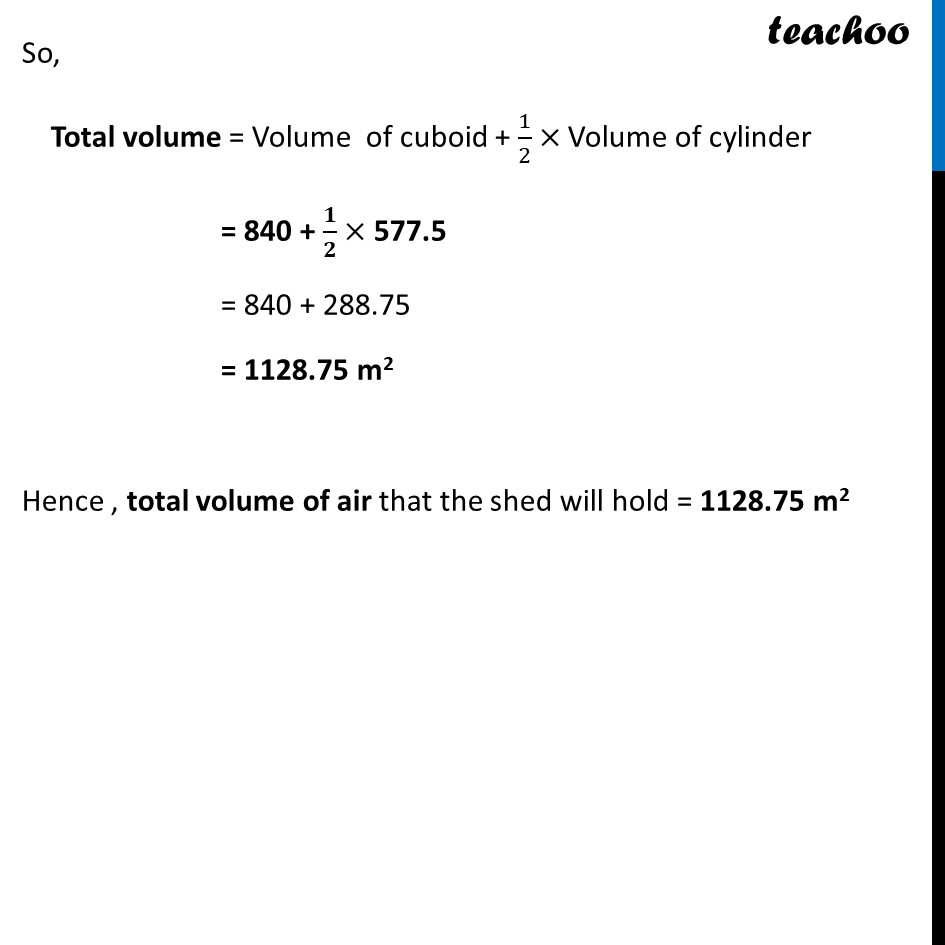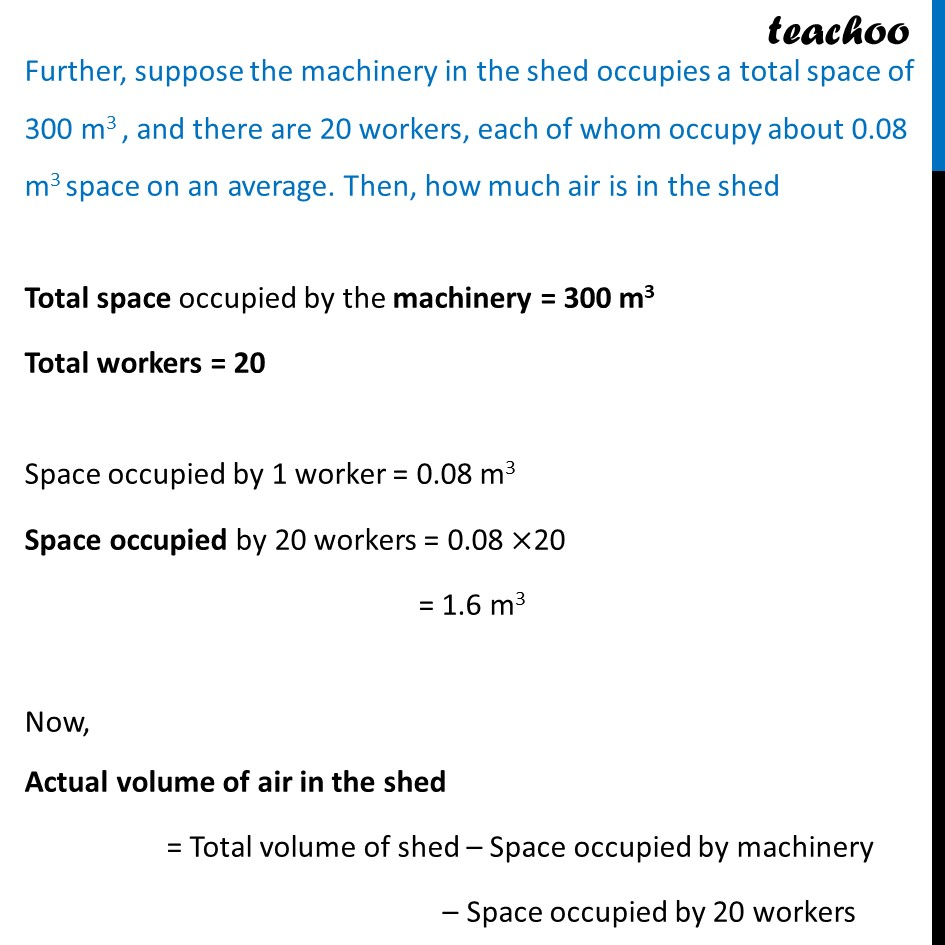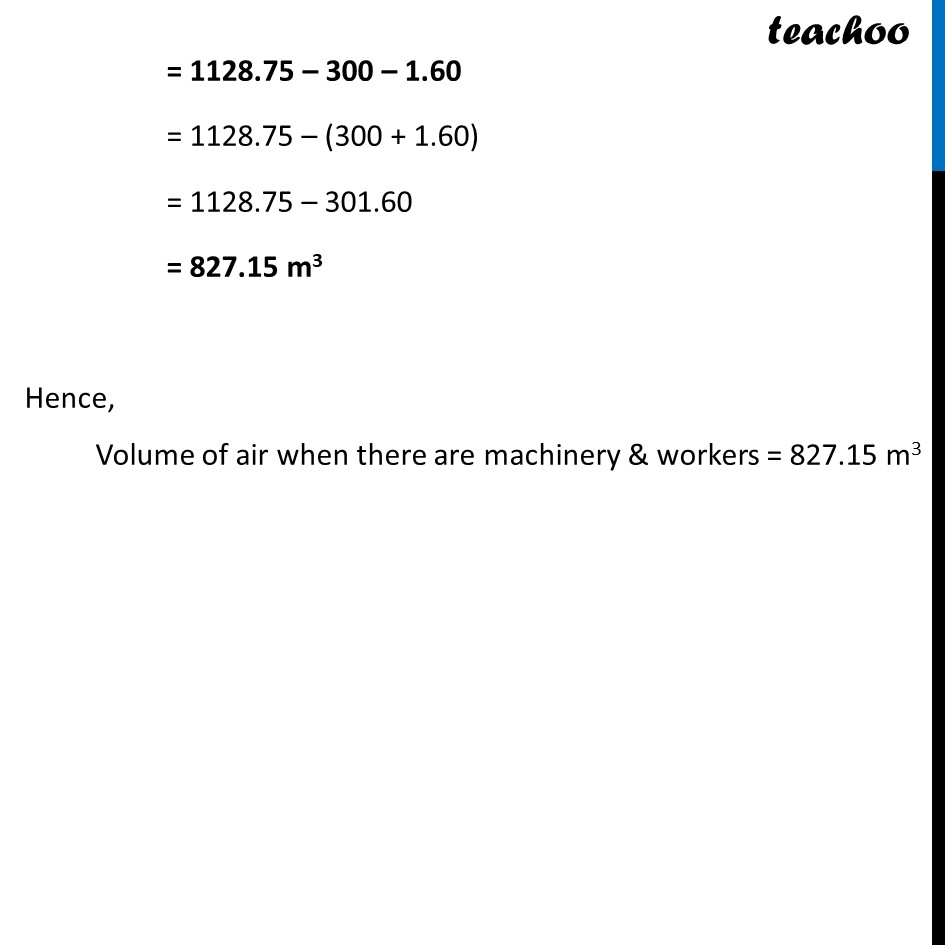Learn in your speed, with individual attention - Teachoo Maths 1-on-1 Class

### Transcript

Example 5 Shanta runs an industry in a shed which is in the shape of a cuboid surmounted by a half cylinder (see figure ). If the base of the shed is of dimension 7 m × 15 m, and the height of the cuboidal portion is 8 m, find the volume of air that the shed can hold. Further, suppose the machinery in the shed occupies a total space of 300 m3 , and there are 20 workers, each of whom occupy about 0.08 m3 space on an average. Then, how much air is in the shed? Take π = 22/7) Now, Total volume = Volume of cuboid + 𝟏/𝟐× Volume of cylinder Volume of cuboid Here, Length = 15 m Breadth = 7m Height = 8 m Volume of cuboid = Length × Bredth × Height = lbh = 15 × 7 × 8 = 840 m2 Volume of cylinder Diameter of cylinder = Breadth of cuboid = 7 m ∴ Radius = 𝐷𝑖𝑎𝑚𝑒𝑡𝑒𝑟/2 = 𝟕/𝟐 m And, Height of cylinder = Length of cuboid = 15 cm Now, Volume of cylinder = 𝜋𝑟2ℎ = 22/7×(7/2)^2 × 15 = 22/7×7/2×7/2×15 = (11 × 7 × 15)/2 = 1155/2 = 577.5 m2 So, Total volume = Volume of cuboid + 1/2× Volume of cylinder = 840 + 𝟏/𝟐× 577.5 = 840 + 288.75 = 1128.75 m2 Hence , total volume of air that the shed will hold = 1128.75 m2 Further, suppose the machinery in the shed occupies a total space of 300 m3 , and there are 20 workers, each of whom occupy about 0.08 m3 space on an average. Then, how much air is in the shed Total space occupied by the machinery = 300 m3 Total workers = 20 Space occupied by 1 worker = 0.08 m3 Space occupied by 20 workers = 0.08 ×20 = 1.6 m3 Now, Actual volume of air in the shed = Total volume of shed – Space occupied by machinery – Space occupied by 20 workers = 1128.75 – 300 – 1.60 = 1128.75 – (300 + 1.60) = 1128.75 – 301.60 = 827.15 m3 Hence, Volume of air when there are machinery & workers = 827.15 m3